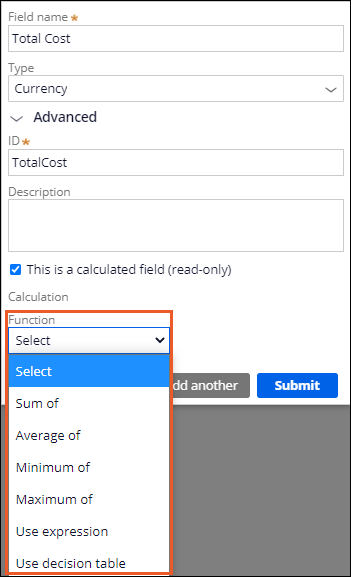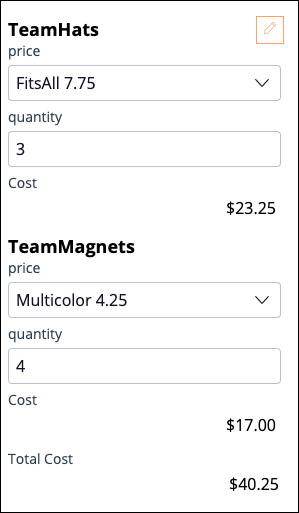# Calculated values

A calculation expresses a relationship between fields by setting the value of a calculated field based on one or more input fields. For example, an online shopping cart application provides customers a total cost for each item ordered, based on unit price and quantity ordered, then calculates a total for all the items ordered.

The application then calculates any assessed tax and adds the tax to the order total to calculate the final charge to the customer. Other calculations can determine the value of promotional discounts and frequent shopper points. Calculation occurs whenever users update the value of an input field. Defining a field calculation reduces the chance for error and improves process efficiency by eliminating the need for a manual calculation.

You can use a calculated field as an input to another calculation, creating a network of dependent calculations. When a user updates the value of a field that serves as a calculation input, the resulting calculation can trigger additional calculations for dependent fields.

App Studio allows three types of calculations:

• Functions iterate over items in a list.
• Expressions calculate a field value by referencing any combination of simple fields, fancy fields, and data relationships.
• Decision tables use a set of conditions to test property values and return an appropriate response.Check your knowledge with the following interaction:

## Functions

Functions iterate over items in a data relationship and are supported for Decimal, Currency, and Integer field types. App Studio provides four basic functions for use in calculations:

• Sum of adds all items in the specified property instances
• Average of calculates the average value of all specified property instances
• Maximum of determines the largest value for all specified property instances
• Minimum of determines the smallest value for all specified property instances

### Sum of

An online retailer needs to calculate a shopping cart total. As customers add items to an order, remove items from an order, or update the quantity of an item on an order, the application must update the total cost. You can use the Sum of function to calculate the Order total field value.

### Average of

An online retailer wants to configure an application dashboard to analyze customer spending patterns so managers can identify the territories that generate the most — and least — revenue on a per-customer basis. You can create a list of submitted orders filtered by territory. You can then use the Average of function to identify the average order value.

### Maximum of

An online retailer needs to allow customers to apply a single promotional discount to an order. Customers submit one or more promotional codes to apply various discounts, such as 20% off the most expensive item or waiving the shipping charge for an order over a specified amount. You can create a promotional discounts data reference, where each item in the list identifies the promotional code and the discount value. You can then use the Maximum of function to determine the most valuable promotional discount to apply to the order.

### Minimum of

An online retailer needs a promotion where preferred customers receive the lowest-cost item from a list of approved free items when placing an order. You can use the Minimum of function to identify the lowest-cost item in the order, and then apply a credit to the order in that amount.

## Expressions

Configure an expression to calculate the field value by using any number of simple fields, fancy fields, or data relationship values. As seen in the following figure, an online ordering application calculates the total cost of the transaction by adding the cost of the TeamHats and the cost of TeamMagnets:Expressions support common operations, such as:

• Subtraction
• Multiplication
• Division
• Grouping (using parenthesis)
• Boolean AND
• Boolean OR

For example, you can configure a calculation to calculate the order tax by multiplying the tax rate by the order total.

.TaxRate * .OrderTotal

You can define an expression for any Simple field type except Email, Phone, and Picklist. For text-based formats, you can configure a calculation to assemble a text string by using a process known as concatenation. For example, an appraisal firm offers to estimate the value of collectible items for customers who submit an item for review. The firm wants to add a comment to the page displaying the item, noting the following information:

• Appraised item name
• Approximate valuation
• Appraiser ID
• Appraisal date

You can define a calculation to assemble the comment by using a combination of field references and static text, where the static text is enclosed with quotation marks (").

"Appraiser " + .AppraiserID + " appraised " + .ItemName + " at a value of " + .AppraisedAmount + " on " + .AppraisalDate + "."

When evaluating the calculation, the application replaces the reference to each field with the current field value. The calculation creates a string similar to the following example:

Appraiser 04795AD appraised Silver platter at a value of USD 350 on January 9, 2019.

Note: In the preceding example, the italicized text indicates the value of a referenced field.

## Decision tables

Decision tables evaluate the value of one or more fields with a set of logical tests to return an appropriate result. For example, to set the interest rate for loans based on the type of loan, the user selects the loan type. The decision table uses the loan type to determine the appropriate interest rate, and the calculation sets the value of the field for rendering in the UI.

Note: A decision table must be marked as a relevant record to show up in the calculated field decision table list. To learn more about marking relevant records, see Marking a record as relevant.

In the center of the following image, slide the vertical line to compare the configuration a System Architect performs in Dev Studio on the left to the configuration a citizen developer performs in App Studio on the right:

## Calculation networks

To identify the relationships between fields, Pega Platform™ establishes and updates a network of calculations for an application. When you define a field calculation, Pega Platform adds that calculation to the calculation network. This calculation network allows Pega Platform to update all relevant fields whenever a value changes. For example, in a shopping cart, changing item quantity can push an update through several dependent calculated fields.

In the following figure, click the + icons to see the functions that trigger the shopping cart calculations:

Check your knowledge with the following interaction: